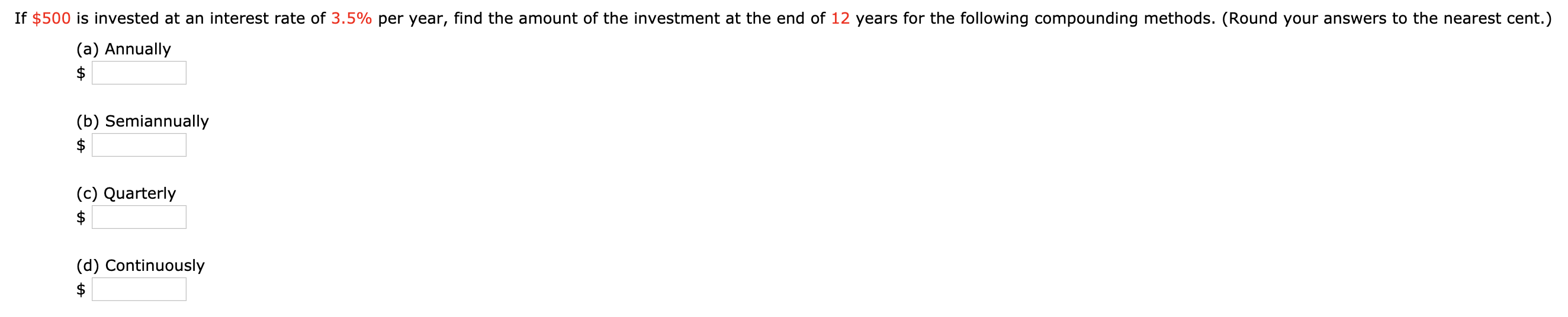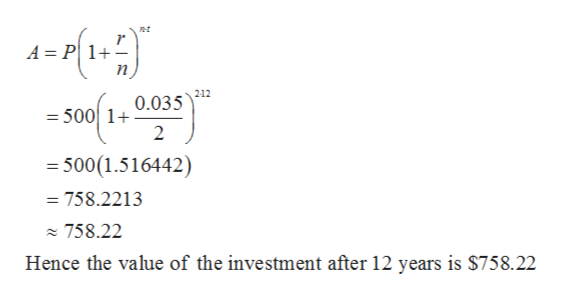# If \$500 is invested at an interest rate of 3.5% per year, find the amount of the investment at the end of 12 years for the following compounding methods. (Round your answers to the nearest cent.)(a) Annually(b) Semiannually\$(c) Quarterly(d) Continuously

Question
29 viewshelp_outlineImage TranscriptioncloseIf \$500 is invested at an interest rate of 3.5% per year, find the amount of the investment at the end of 12 years for the following compounding methods. (Round your answers to the nearest cent.) (a) Annually (b) Semiannually \$ (c) Quarterly (d) Continuously fullscreen
check_circle

Step 1

Given,

Step 2

a). t = 12 years and n=1 [because compounded annually]

Therefore

Step 3

b). n = 2 [because compounded sem...help_outlineImage Transcriptioncloset A = P 1+ n 212 0.035 = 500 1+ 2 500(1.516442) = 758.2213 758.22 Hence the value of the investment after 12 years is \$758.22 fullscreen

### Want to see the full answer?

See Solution

#### Want to see this answer and more?

Solutions are written by subject experts who are available 24/7. Questions are typically answered within 1 hour.*

See Solution
*Response times may vary by subject and question.
Tagged in

### Other